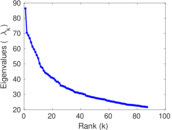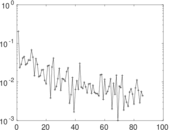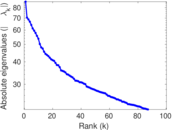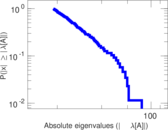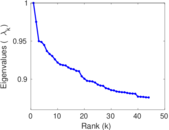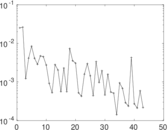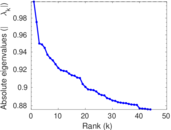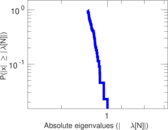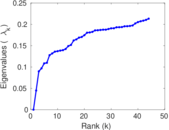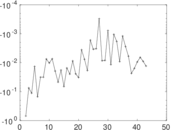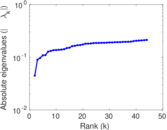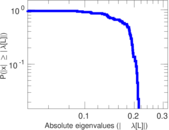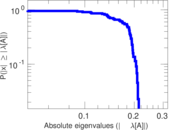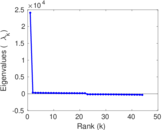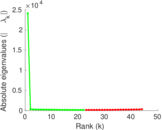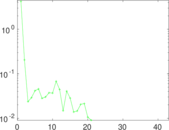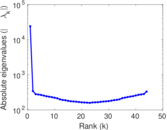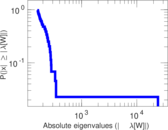# MovieLens tag–item

This bipartite network consists of 100,000 tags applied to 10,000 movies from http://movielens.umn.edu/. Left nodes are tags and right nodes are movies. An edge shows that a tag was applied to a movie.

 Code `Mti` Internal name `movielens-10m_ti` Name MovieLens tag–item Data source http://www.grouplens.org/node/73 AvailabilityDataset is available for download Consistency checkDataset passed all tests Category Feature network Node meaning Tag, movie Edge meaning Tag assignment Network formatBipartite, undirected Edge typeUnweighted, multiple edges Temporal dataEdges are annotated with timestamps

## Statistics

 Size n = 24,129 Left size n1 = 16,528 Right size n2 = 7,601 Volume m = 95,580 Unique edge count m̿ = 71,154 Wedge count s = 3,229,651 Claw count z = 205,226,828 Cross count x = 18,155,774,783 Square count q = 959,491 4-Tour count T4 = 20,740,224 Maximum degree dmax = 641 Maximum left degree d1max = 641 Maximum right degree d2max = 308 Average degree d = 7.922 42 Average left degree d1 = 5.782 91 Average right degree d2 = 12.574 7 Fill p = 0.000 566 380 Average edge multiplicity m̃ = 1.343 28 Size of LCC N = 23,760 Diameter δ = 14 50-Percentile effective diameter δ0.5 = 4.282 43 90-Percentile effective diameter δ0.9 = 5.690 08 Median distance δM = 5 Mean distance δm = 4.781 08 Gini coefficient G = 0.743 230 Balanced inequality ratio P = 0.202 307 Left balanced inequality ratio P1 = 0.198 347 Right balanced inequality ratio P2 = 0.250 983 Relative edge distribution entropy Her = 0.894 530 Power law exponent γ = 2.122 38 Tail power law exponent γt = 3.141 00 Tail power law exponent with p γ3 = 3.141 00 p-value p = 0.170 000 Left tail power law exponent with p γ3,1 = 1.981 00 Left p-value p1 = 0.000 00 Right tail power law exponent with p γ3,2 = 4.201 00 Right p-value p2 = 0.067 000 0 Degree assortativity ρ = −0.085 398 3 Degree assortativity p-value pρ = 2.834 24 × 10−115 Spectral norm α = 86.440 1 Algebraic connectivity a = 0.044 767 2 Spectral separation |λ1[A] / λ2[A]| = 1.226 55 Controllability C = 12,418 Relative controllability Cr = 0.514 650

## Plots

### Fruchterman–Reingold graph drawing### Degree distribution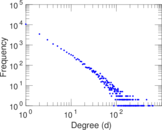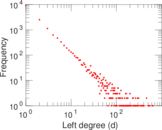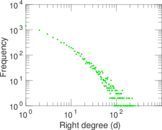### Cumulative degree distribution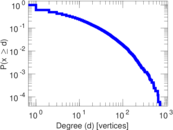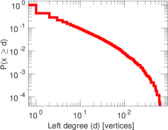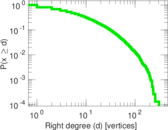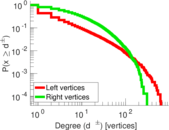### Lorenz curve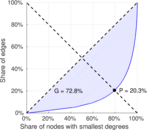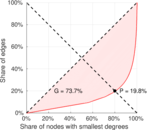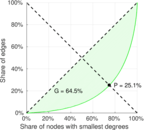### Spectral distribution of the adjacency matrix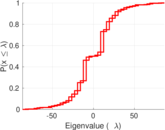### Spectral distribution of the normalized adjacency matrix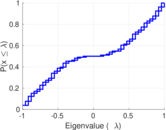### Spectral distribution of the Laplacian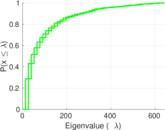### Spectral graph drawing based on the adjacency matrix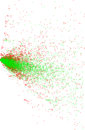### Spectral graph drawing based on the Laplacian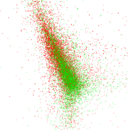### Spectral graph drawing based on the normalized adjacency matrix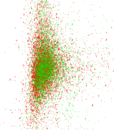### Degree assortativity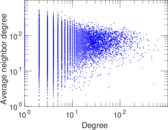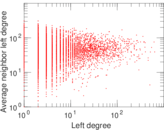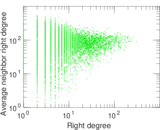### Zipf plot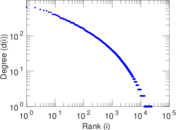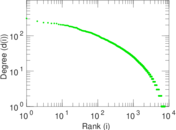### Hop distribution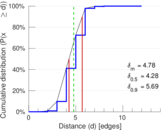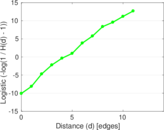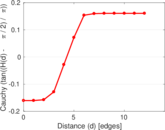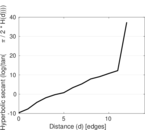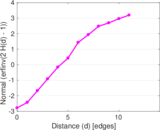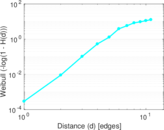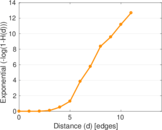### Double Laplacian graph drawing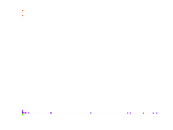### Delaunay graph drawing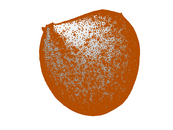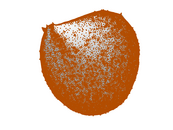### Edge weight/multiplicity distribution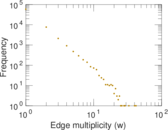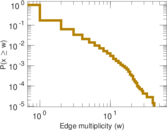### Temporal distribution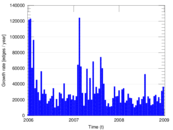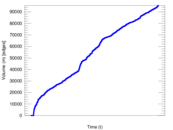### Temporal hop distribution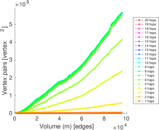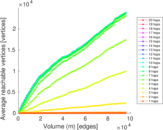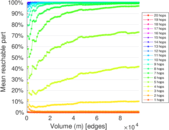### Diameter/density evolution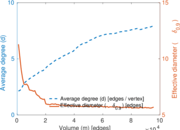### Inter-event distribution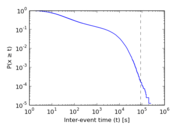### Node-level inter-event distribution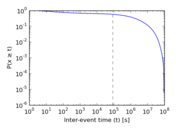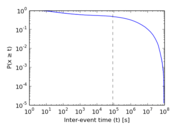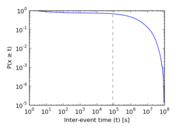### Matrix decompositions plots